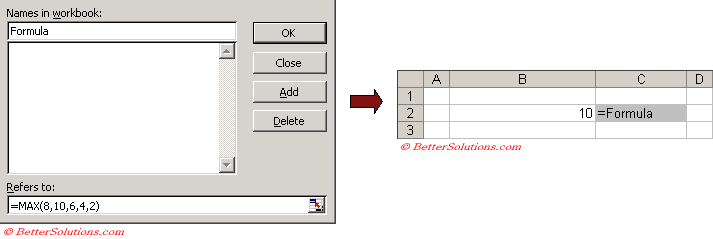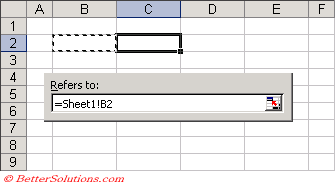# Naming Formulas

All the arguments to the formula must be static.
You can use a named range formula anywhere you can use a normal formula.
It is even possible to create a named range formula that refers to a range of cells where the range varies depending on the contents of the cells. This is sometimes called a dynamic named range.
You can enter a formula directly into the "refers to" box. Type "=" followed by the formula.
You can also use a named range to enter a formula directly. The formula that you enter uses the active cell references "relative" to the active cell (i.e. the cell that receives the formula).
The formula that you enter uses the cell references relative to the active cell (i.e. the cell containing the formula).To use a named range formula in a cell, select the cell, press F2 to edit the cell.
Select (Insert > Name > Paste) and select the name.

### Naming Relative Formulas

Whenever you create a named range to refer to a cell or range of cells absolute cell references are used.
Selecting cell "D2" and typing a name directly into the Name box to the right of the formula bar is just a shortcut for entering a named range which refers to "=\$D\$2".
Likewise selecting a cell range "D2:E10" is just a shortcut for entering a named range which refers to "=\$D\$2:\$E\$10".
The formula that you enter will use relative references to the active cell.
If you use the mouse to select the related cells the references will be absolute.
It is possible to use relative cell references as well by overwriting the reference in the (Insert > Name > Define) dialog box.
You can create a named range that always refers to the cell directly to the left by selecting any cell, and in the refers to box select the cell immediately to the left. The important step is to remove the absolute references "\$" from the cell reference.To test the relative named formula just type a value into a cell and the type "=Cell_Left" into the cell directly to its left.
Excel will update this formula every time the value in the cell on the left changes.
Remember that for this function to work, the refers to reference must be the cell immediately to the left of the cell that is currently selected.
You can also combine absolute and mixed cell references in your named formulas and also use worksheet functions.

### Important

Any named constants or formulas will not appear in the Name box to the left of the formula bar.
Any constant named ranges or formula named ranges will not appear in the Name box or in the (Edit > GoTo) dialog box. They will only appear in the (Insert > Name > Define) dialog box.